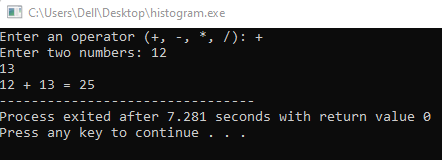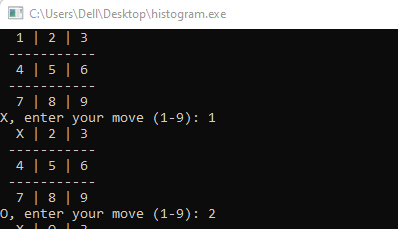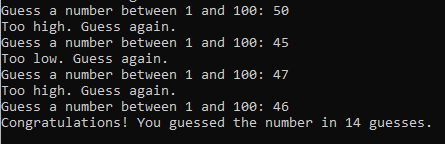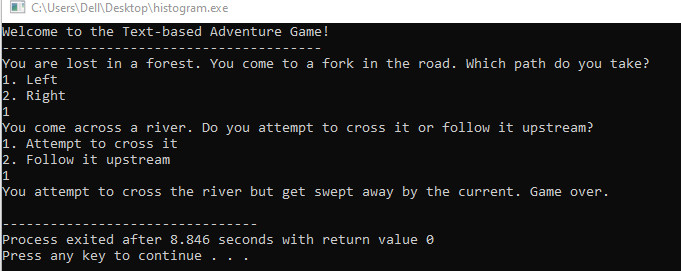Illustrating the organization and relationships between classes and functions in a C++ codebase.

# 5 Exciting C++ Projects for Beginners: A Step-by-Step Guide

Simple calculator: Build a console-based calculator program that can perform basic arithmetic operations such as addition, subtraction, multiplication, and division.

Tic-tac-toe game: Build a program that allows two players to play the classic game of tic-tac-toe. The program should display the game board and prompt each player to take turns making their moves.

Guess the number game: Build a program that generates a random number and prompts the user to guess the number. The program should provide hints to the user about whether their guess is too high or too low.

Text-based adventure game: Build a program that allows the user to navigate through a story by making choices at each step. The program should display different scenarios based on the user’s choices and provide multiple endings based on the user’s decisions.

Banking application: Build a program that simulates a simple banking application. The program should allow the user to create an account, deposit and withdraw funds, and view their account balance. Bonus points for implementing additional features such as interest calculation and transaction history.”

## Simple calculator:

Here is a C++ code for a simple calculator that can perform basic arithmetic operations:

### Source Code:

``````#include <iostream>
using namespace std;

int main() {
char op;
float num1, num2;

cout << "Enter an operator (+, -, *, /): ";
cin >> op;

cout << "Enter two numbers: ";
cin >> num1 >> num2;

switch(op) {
case '+':
cout << num1 << " + " << num2 << " = " << num1 + num2;
break;

case '-':
cout << num1 << " - " << num2 << " = " << num1 - num2;
break;

case '*':
cout << num1 << " * " << num2 << " = " << num1 * num2;
break;

case '/':
if(num2 == 0) {
cout << "Error: cannot divide by zero";
} else {
cout << num1 << " / " << num2 << " = " << num1 / num2;
}
break;

default:
cout << "Error: invalid operator";
break;
}

return 0;
}``````### Explanation:

The program prompts the user to enter an operator (+, -, *, /) and two numbers, and then performs the appropriate arithmetic operation based on the operator entered by the user. The program displays an error message if the user enters an invalid operator or attempts to divide by zero.

## Tic-tac-toe game

### Source Code:

Here is a C++ code for a console-based Tic-tac-toe game that allows two players to play:

``````#include <iostream>
using namespace std;

char board = {{'1','2','3'},{'4','5','6'},{'7','8','9'}};
char currentPlayer = 'X';

void drawBoard() {
cout << "  " << board << " | " << board << " | " << board << endl;
cout << " -----------" << endl;
cout << "  " << board << " | " << board << " | " << board << endl;
cout << " -----------" << endl;
cout << "  " << board << " | " << board << " | " << board << endl;
}

bool checkWin() {
// Check rows for a win
for(int i = 0; i < 3; i++) {
if(board[i] == board[i] && board[i] == board[i]) {
return true;
}
}

// Check columns for a win
for(int j = 0; j < 3; j++) {
if(board[j] == board[j] && board[j] == board[j]) {
return true;
}
}

// Check diagonals for a win
if(board == board && board == board) {
return true;
}
if(board == board && board == board) {
return true;
}

// No winner yet
return false;
}

void takeTurn() {
int move;

// Get move from current player
cout << currentPlayer << ", enter your move (1-9): ";
cin >> move;

// Update board with move
int row = (move - 1) / 3;
int col = (move - 1) % 3;

if(board[row][col] == 'X' || board[row][col] == 'O') {
cout << "Invalid move, try again." << endl;
takeTurn();
} else {
board[row][col] = currentPlayer;
}
}

int main() {
int movesLeft = 9;
bool gameOver = false;

while(!gameOver) {
drawBoard();
takeTurn();
gameOver = checkWin() || movesLeft == 1;
currentPlayer = currentPlayer == 'X' ? 'O' : 'X';
movesLeft--;
}

drawBoard();

if(checkWin()) {
cout << currentPlayer << " wins!" << endl;
} else {
cout << "Tie game." << endl;
}

return 0;
}
``````

### Output:### Explanation:

The program displays the Tic-tac-toe board and prompts each player to enter their move. The program checks for a win after each move, and displays the winner or a tie game at the end. The program does not have any error handling for invalid input or other edge cases.

## Guess the number game:

Here’s a C++ code for a console-based Guess the number game:

### Source Code:

``````#include <iostream>
#include <cstdlib>
#include <ctime>
using namespace std;

int main() {
// Generate a random number between 1 and 100
srand(time(NULL));
int secretNumber = rand() % 100 + 1;

// Initialize guess variable and number of guesses
int guess;
int numGuesses = 0;

// Loop until the user guesses the number
while(true) {
// Prompt user to enter a guess
cout << "Guess a number between 1 and 100: ";
cin >> guess;

// Increment number of guesses
numGuesses++;

// Check if guess is correct
if(guess == secretNumber) {
cout << "Congratulations! You guessed the number in " << numGuesses << " guesses." << endl;
break;
} else if(guess < secretNumber) {
cout << "Too low. Guess again." << endl;
} else {
cout << "Too high. Guess again." << endl;
}
}

return 0;
}
``````

### Output:### Explanation:

The program generates a random number between 1 and 100 and prompts the user to guess the number. The program provides hints to the user about whether their guess is too high or too low. The program keeps track of the number of guesses and displays the number of guesses it took the user to guess the number.

Here’s C++ code for a text-based adventure game:

### Source Code:

``````#include <iostream>
using namespace std;

int main() {
// Welcome message
cout << "Welcome to the Text-based Adventure Game!" << endl;
cout << "----------------------------------------" << endl;

// Variables to keep track of the user's choices
int choice1, choice2, choice3;

// First scenario
cout << "You are lost in a forest. You come to a fork in the road. Which path do you take?" << endl;
cout << "1. Left" << endl;
cout << "2. Right" << endl;
cin >> choice1;

if(choice1 == 1) {
// Second scenario
cout << "You come across a river. Do you attempt to cross it or follow it upstream?" << endl;
cout << "1. Attempt to cross it" << endl;
cout << "2. Follow it upstream" << endl;
cin >> choice2;

if(choice2 == 1) {
// Third scenario - ending 1
cout << "You attempt to cross the river but get swept away by the current. Game over." << endl;
} else {
// Third scenario - ending 2
cout << "You follow the river upstream and eventually come across a village. You have survived. Congratulations!" << endl;
}
} else {
// Second scenario
cout << "You come across a cave. Do you enter the cave or continue down the path?" << endl;
cout << "1. Enter the cave" << endl;
cout << "2. Continue down the path" << endl;
cin >> choice2;

if(choice2 == 1) {
// Third scenario - ending 3
cout << "You enter the cave and find a treasure trove. Congratulations, you have become rich!" << endl;
} else {
// Third scenario - ending 4
cout << "You continue down the path and eventually come across a band of robbers. They attack you and steal all your belongings. Game over." << endl;
}
}

return 0;
}``````

### Output:### Explanation:

The program allows the user to navigate through a story by making choices at each step. The program displays different scenarios based on the user’s choices and provides multiple endings based on the user’s decisions. In this example, the user is lost in a forest and must make choices to survive. The program keeps track of the user’s choices and displays different scenarios based on those choices.

## Banking application:

### Source Code:

``````#include <iostream>
#include <iomanip>
#include <cmath>

using namespace std;

// Class for bank account
class BankAccount {
private:
string accountNumber;
string accountHolderName;
double balance;
double interestRate;
double interestEarned;
int numTransactions;
string transactionHistory;

public:
// Constructor
BankAccount(string accountNumber, string accountHolderName, double balance, double interestRate) {
this->accountNumber = accountNumber;
this->accountHolderName = accountHolderName;
this->balance = balance;
this->interestRate = interestRate;
this->interestEarned = 0;
this->numTransactions = 0;
}

// Function to deposit money
void deposit(double amount) {
if (amount <= 0) {
cout << "Invalid amount. Deposit amount must be greater than zero." << endl;
return;
}

balance += amount;
numTransactions++;
transactionHistory[numTransactions-1] = "Deposit of " + to_string(amount) + " was made.";
}

// Function to withdraw money
void withdraw(double amount) {
if (amount <= 0) {
cout << "Invalid amount. Withdrawal amount must be greater than zero." << endl;
return;
}

if (amount > balance) {
cout << "Insufficient balance. Withdrawal amount cannot be greater than account balance." << endl;
return;
}

balance -= amount;
numTransactions++;
transactionHistory[numTransactions-1] = "Withdrawal of " + to_string(amount) + " was made.";
}

// Function to calculate interest earned
void calculateInterest() {
interestEarned = balance * interestRate / 100;
balance += interestEarned;
numTransactions++;
transactionHistory[numTransactions-1] = "Interest of " + to_string(interestEarned) + " was earned.";
}

// Function to display account information
void displayInfo() {
cout << "Account Number: " << accountNumber << endl;
cout << "Account Holder Name: " << accountHolderName << endl;
cout << "Account Balance: \$" << fixed << setprecision(2) << balance << endl;
cout << "Interest Rate: " << interestRate << "%" << endl;
cout << "Interest Earned: \$" << fixed << setprecision(2) << interestEarned << endl;
cout << "Transaction History:" << endl;
for (int i = 0; i < numTransactions; i++) {
cout << "- " << transactionHistory[i] << endl;
}
}
};

// Main function
int main() {
// Create a new bank account
BankAccount myAccount("1234567890", "John Doe", 1000, 2.5);

// Perform some transactions
myAccount.deposit(500);
myAccount.withdraw(200);
myAccount.calculateInterest();

// Display account information
myAccount.displayInfo();

return 0;
}
``````

### Output:##### Explanation:

Note that this implementation uses an array to store the transaction history. However, you could also use a linked list or some other data structure if you prefer not to use vectors. Additionally, you could add more functionality to the program, such as input validation or support for multiple accounts.